# Qlik Sense App Development

Announcements
Make your voice heard! Participate in the 2020 Wisdom of Crowds® Survey. BEGIN SURVEY
HighlightedPartner

## If Count Expression

Hello!

I don't know what I'm doing wrong with the following expression.  I'm probably not using the parenthesis correctly.  I've used this exact expression before, just without the count portion.

This time, I'm adding the count portion to the expression.  If a [filename] has a count of 0-10, then '0-10 Times', etc.

=

If(count[filename]<=10,Dual('0-10 Times',0),

If(count[filename]>10 and count[filename] <=30,Dual('11-30 Times',1),

If(count[filename]>30 and count[filename] <=50,Dual('31-50 Times',2),

If(count[filename]>50,Dual('50+ Times',3),

))))

1 Solution

Accepted Solutions
HighlightedMVP

## Re: If Count Expression

So, this was getting used as a calculated dimension... try this

=Aggr(

If(count([filename]) <= 10, Dual('0-10 Times', 0),

If(count([filename]) > 10 and count([filename]) <= 30, Dual('11-30 Times', 1),

If(count([filename]) > 30 and count([filename]) <= 50, Dual('31-50 Times', 2),

If(count([filename]) > 50, Dual('50+ Times', 3)))))

, [filename])

12 RepliesMVP

## Re: If Count Expression

Is this by any chance used as a calculated dimension or a list box expression? If not, then may be try this

=If(count([filename]) <= 10, Dual('0-10 Times', 0),

If(count([filename]) > 10 and count([filename]) <= 30, Dual('11-30 Times', 1),

If(count([filename]) > 30 and count([filename]) <= 50, Dual('31-50 Times', 2),

If(count([filename]) > 50, Dual('50+ Times', 3)))))

HighlightedPartner

## Re: If Count Expression

Hi Lea,

You missed ()

=If(count([filename]) <= 10, Dual('0-10 Times', 0),

If(count([filename]) > 10 and count([filename]) <= 30, Dual('11-30 Times', 1),

If(count([filename]) > 30 and count([filename]) <= 50, Dual('31-50 Times', 2),

If(count([filename]) > 50, Dual('50+ Times', 3)))))

Thanks,

Arvind Patil

HighlightedPartner

## Re: If Count Expression

Hi Sunny,

It did not work.  The expression was marked as "OK", but the dimension is showing as "Invalid":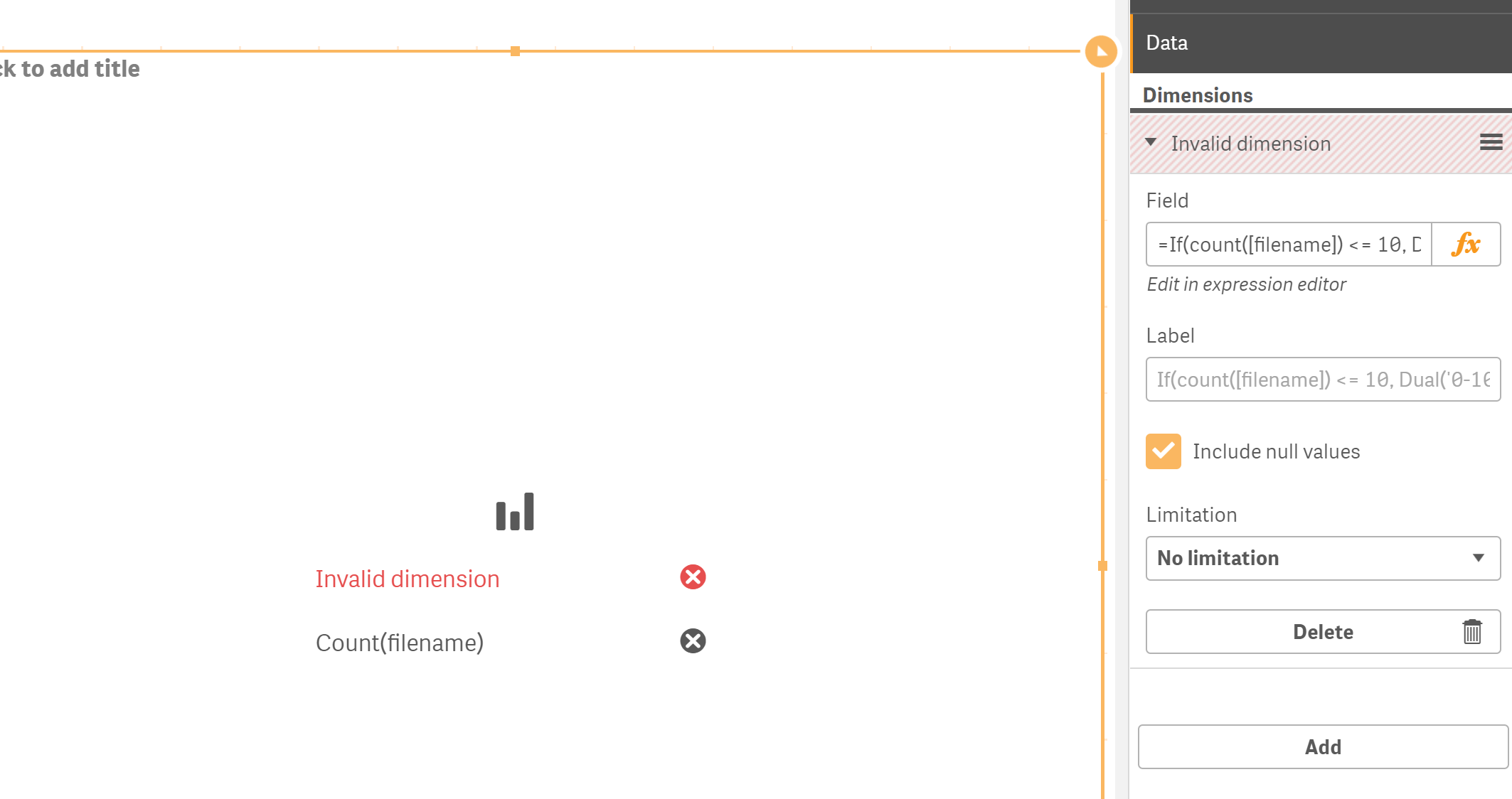To give you some more background on what I'm trying to do, I want to create a bar chart similar to this one:where the count of the [filename] is grouped into 1-10 Times, 11-30 Times, etc.

HighlightedPartner

## Re: If Count Expression

Hi Arvind,

It did not work.  Please see my response to Sunny above.  Do you have any suggestions?

HighlightedMVP

## Re: If Count Expression

So, this was getting used as a calculated dimension... try this

=Aggr(

If(count([filename]) <= 10, Dual('0-10 Times', 0),

If(count([filename]) > 10 and count([filename]) <= 30, Dual('11-30 Times', 1),

If(count([filename]) > 30 and count([filename]) <= 50, Dual('31-50 Times', 2),

If(count([filename]) > 50, Dual('50+ Times', 3)))))

, [filename])

HighlightedPartner

## Re: If Count Expression

Yes, it worked.

Thanks so much!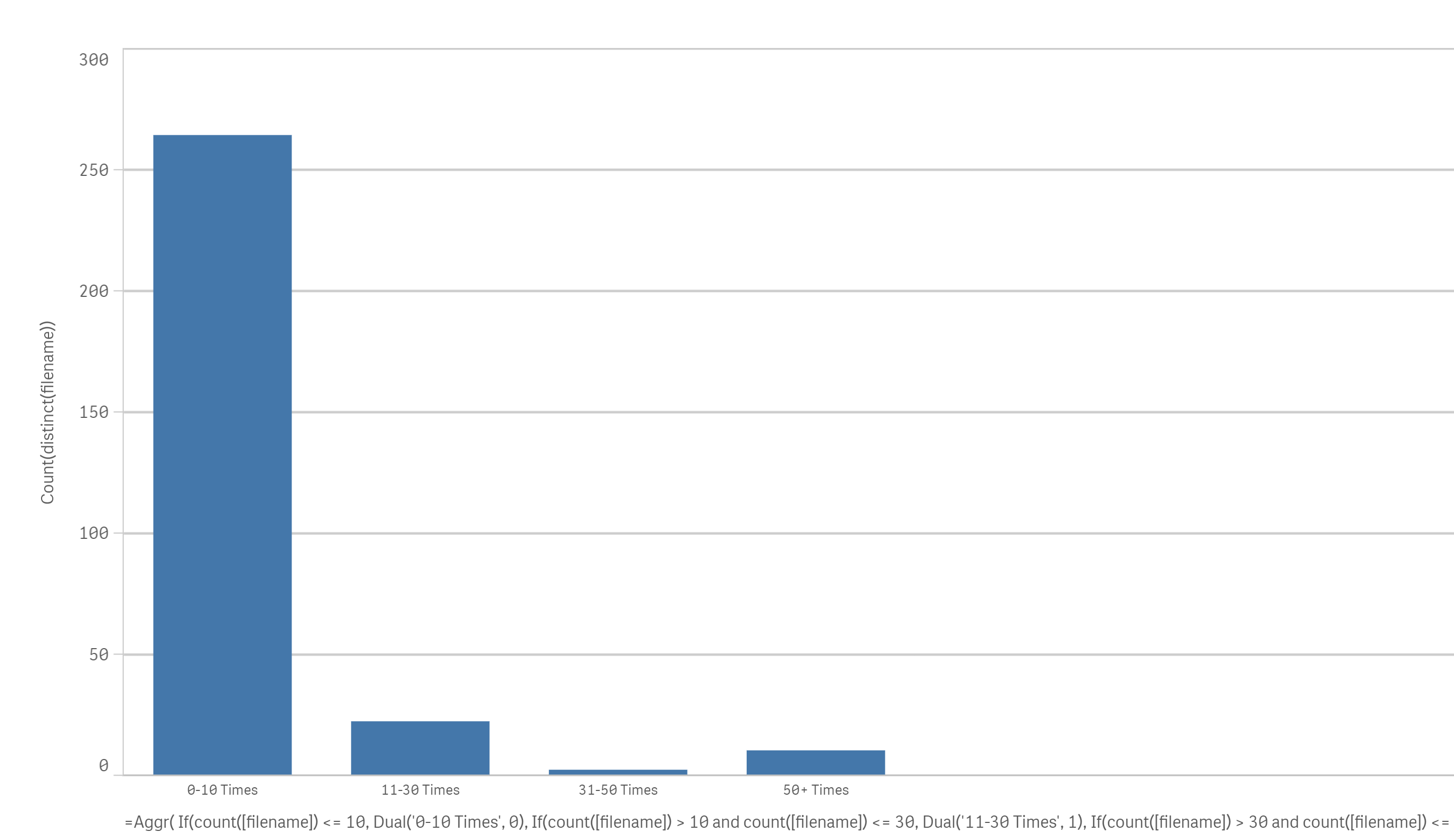HighlightedPartner

## Re: If Count Expression

Hi Sunny,

I need additional help with this formula.  I have this bar chart that I created with the formula that you provided:Now, if I make a selection and on this bar chart, for lets say, 31-50 Times, this works.  I get this result: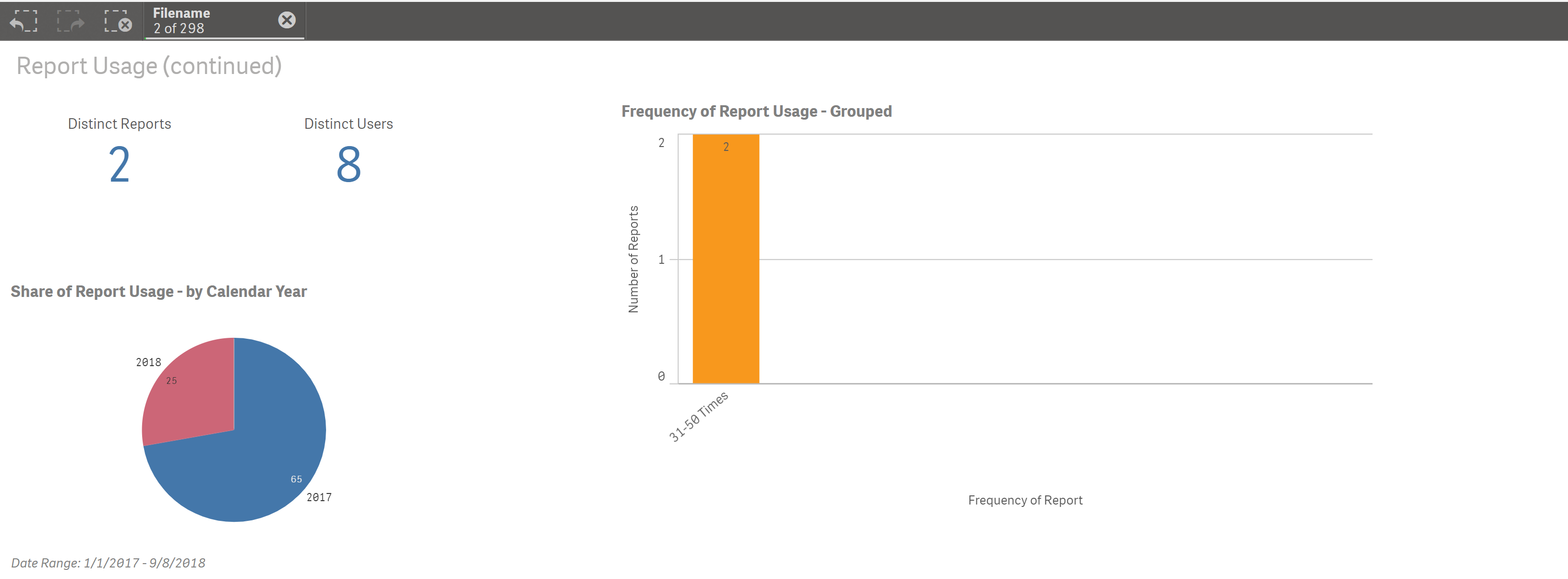But now if I make a  selection on the pie chart, for lets say, 2018, the bar chart changes: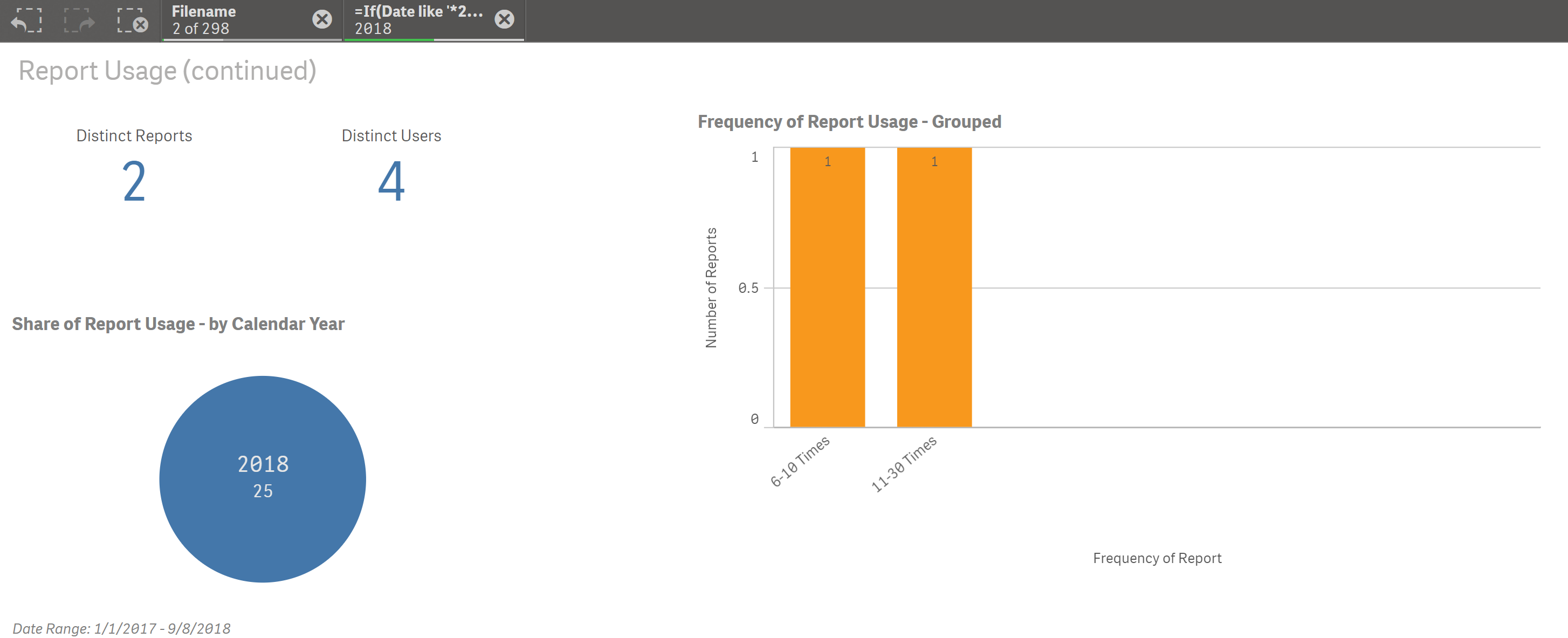Instead of giving me these results, I would want there to be no columns shown on the bar chart because there are no longer any filenames in 2018 that were used 31-50 Times.

I understand why it's giving me these results - because we actually filtered for the "Filename" and not for "31-50 Times":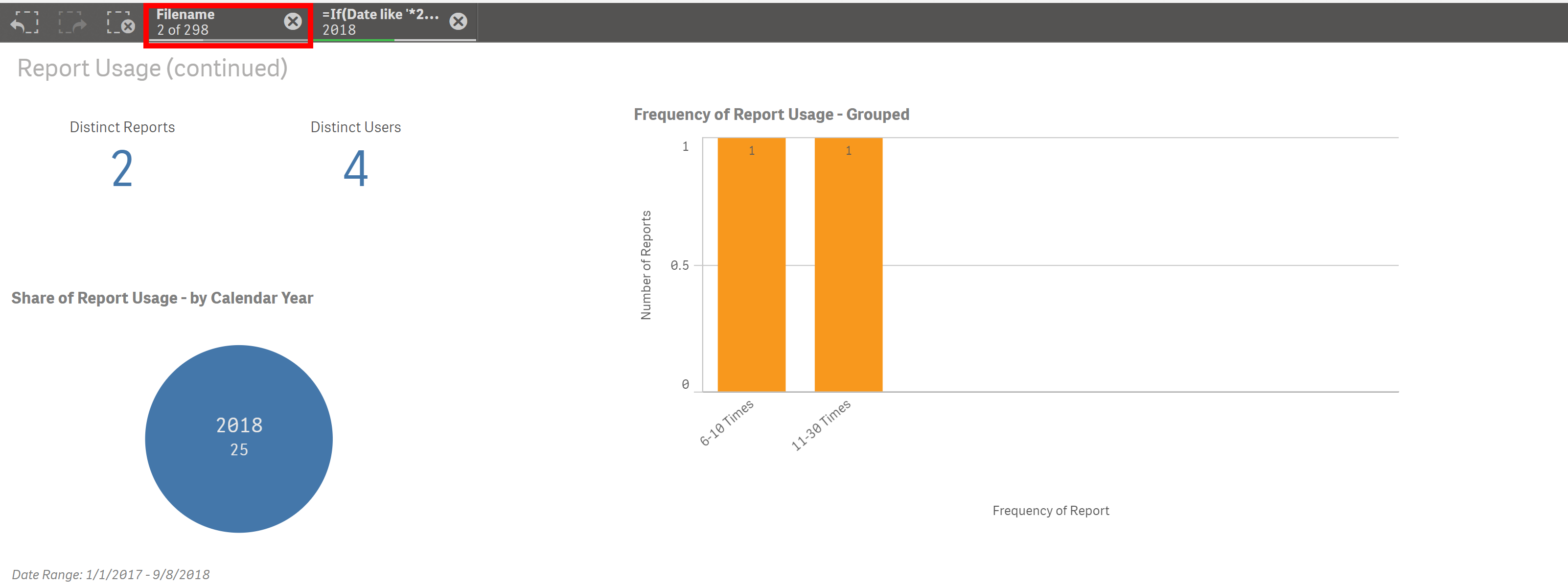How can I adjust the formula that you gave me earlier to fix this problem?

Thank you!

HighlightedMVP

## Re: If Count Expression

Try this

=Aggr(

If(count({<Year>} [filename]) <= 10, Dual('0-10 Times', 0),

If(count({<Year>} [filename]) > 10 and count({<Year>} [filename]) <= 30, Dual('11-30 Times', 1),

If(count({<Year>} [filename]) > 30 and count({<Year>} [filename]) <= 50, Dual('31-50 Times', 2),

If(count({<Year>} [filename]) > 50, Dual('50+ Times', 3)))))

, [filename])

HighlightedPartner

## Re: If Count Expression

No, that gave me this result:And I'll clarify that the problem doesn't just happen when I select a specific Year.  It also happens when I make a selection on any other field.